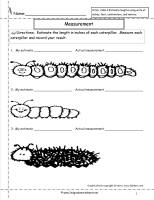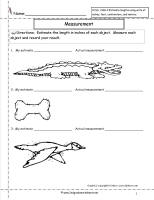﻿ CCSS 2.MD.3 Worksheets, Estimating and Measuring Lengths Worksheets

# Common Core State Standard 2.MD.3

## Measurement & Data

### Measure and estimate lengths in standard units.

Estimate lengths using units of inches, feet, centimeters, and meters.

###Estimate and Measure Worksheet

Common Core State Standards: 2.MD.3
Measurement & Data
Measure and estimate lengths in standard units.

Estimate lengths using units of inches, feet, centimeters, and meters.

Students estimate length and measure in inches.

###Estimate and Measure Worksheet

Common Core State Standards: 2.MD.3
Measurement & Data
Measure and estimate lengths in standard units.

Estimate lengths using units of inches, feet, centimeters, and meters.

Students estimate length and measure in inches.

###Estimate and Measure Worksheet

Common Core State Standards: 2.MD.3
Measurement & Data
Measure and estimate lengths in standard units.

Estimate lengths using units of inches, feet, centimeters, and meters.

Students estimate length and measure in centimeters.

###Estimate and Measure Worksheet

Common Core State Standards: 2.MD.3
Measurement & Data
Measure and estimate lengths in standard units.

Estimate lengths using units of inches, feet, centimeters, and meters.

Students estimate length and measure in centimeters.